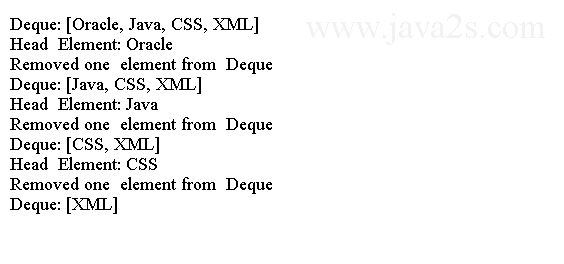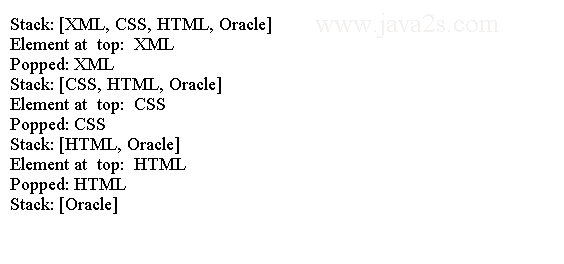# Java Collection Tutorial - Java Double Ended Queues

A doubly ended queue or deque extends a queue to allow insertion and removal of elements from both ends.

An instance of `Deque` class represents a doubly ended queue. The `Deque` interface extends the `Queue` interface.

It declares additional methods to facilitate all the operations for a queue at the head as well as at the tail. It can be used as a FIFO queue or a LIFO queue.

The `ArrayDeque` and `LinkedList` classes are two implementation classes for the Deque interface.

The `ArrayDeque` class is backed by an array whereas the `LinkedList` class is backed by a linked list.

You should use the `ArrayDeque` as a `Deque` implementation if you are using a Deque as a stack.

The `LinkedList` implementation performs better if you use a `Deque` as a FIFO queue.

The following code shows how to use a `Deque` as a FIFO Queue.

```import java.util.Deque;
/*from   w ww.j  a va 2  s . co m*/
public class Main {
public static void main(String[] args) {
deque.offerLast("Java");
deque.offerLast("CSS");
deque.offerLast("XML");

System.out.println("Deque: " + deque);

// remove elements from the Deque until it is empty
while (deque.peekFirst() != null) {
deque.removeFirst();
System.out.println("Removed one  element from  Deque");
System.out.println("Deque: " + deque);
}

// the Deque is empty. Try to call its peekFirst(),
// getFirst(), pollFirst() and removeFirst() methods
System.out.println("deque.isEmpty(): " + deque.isEmpty());

System.out.println("deque.peekFirst(): " + deque.peekFirst());
System.out.println("deque.pollFirst(): " + deque.pollFirst());

String str = deque.getFirst();
System.out.println("deque.getFirst(): " + str);
str = deque.removeFirst();
System.out.println("deque.removeFirst(): " + str);

}
}
```

The code above generates the following result.## Example

The following code shows how to use a Deque as a stack (or LIFO queue).

```import java.util.ArrayDeque;
import java.util.Deque;
/*from www.j a va2  s  .c  om*/
public class Main {
public static void main(String[] args) {
// Create a Deque and use it as stack
Deque<String> deque = new ArrayDeque<>();
deque.push("Oracle");
deque.push("HTML");
deque.push("CSS");
deque.push("XML");

System.out.println("Stack: " + deque);

// remove all elements from the Deque
while (deque.peek() != null) {
System.out.println("Element at  top:  " + deque.peek());
System.out.println("Popped: " + deque.pop());
System.out.println("Stack: " + deque);
}

System.out.println("Stack is  empty:  " + deque.isEmpty());
}
}
```

The code above generates the following result.